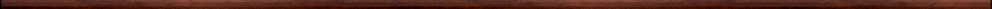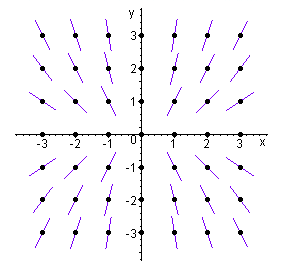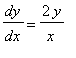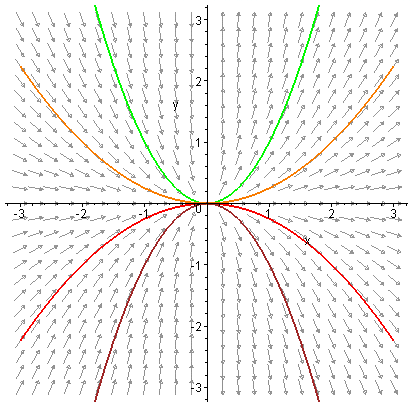Maple worksheets on first order differential equationsDifferential equations:

They are all compatible with Classic Worksheet Maple 10.Introduction to differential equations - DEintro.mws

• What is a differential equation?
• Preliminary examples.
• The direction, or gradient field, associated with a differential equation.
• Solving differential equations with Maple using dsolve.
• Plotting a solution of a differential equation.
• Plotting a solution along with the gradient field.
• Using dsolve( . . , type=numeric) and odeplot.

First order differential equations with separable variables I - sepvar.mws

• General method of solving first order DE's with separable variables.
• Examples

More examples of 1st order differential equations with separable variables - sepvar2.mws

A procedure for solving 1st order differential equations with separable variables - sepvar3.mws

• A procedure for solving first order DE's with separable variables - desolveSV.
• General solution examples
• Particular solution examples
• A symbolic example

First order linear differential equations - linearDE.mws

• Method of solution of first order linear DE's
• The formula for the Integrating factor

A procedure for solving 1st order linear differential equations - linearDE2.mws

• A procedure for solving first order linear DE's - desolveLN.
• General solution examples
• Particular solution examples

A procedure for solving 1st order homogeneous differential equations - homogDE.mws

• Solving first order homogeneous DE's.
• A procedure for solving first order homogeneous DE's - desolveHG.
• General solution examples
• Particular solution examples

First order exact differential equations - exactDE.mws

• What is an exact differential equation?
• Method of solution of exact differential equations.
• Graphing implicit solutions of an exact DE.
• Alternative scheme for solving exact DE's.

A procedure for solving 1st order exact differential equations - exactDE2.mws

• A procedure for solving first order exact DE's: desolveEX.
• General solution examples
• Particular solution examples

Applications of first order differential equations - appDE.mws

• An RL circuit
• Population growth - the logistic equation
• An object falling in a resisting medium
• An RC circuit
• The rate of a chemical reaction

First order Bernoulli differential equations - bernoulliDE.mws

• First order Bernoulli DE's.
• A procedure for solving first order Bernoulli DE's: desolveBN.
• Examples.

First order inverse linear differential equations - invlinearDE.mws

• First order inverse linear DE's.
• A procedure for solving first order inverse linear DE's: desolveNL.
• Examples.

Procedures for differential equations - DEsol.zip

Top of page

Main index